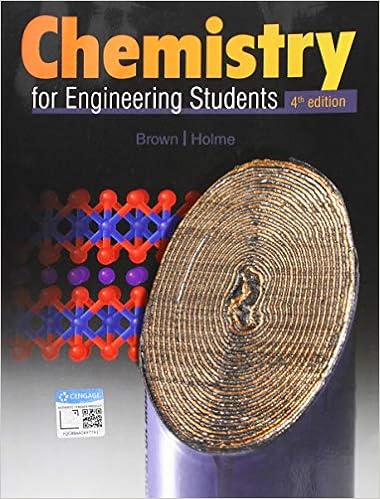Given the values of h rxn s rxn and t below determine

• Homework Help
• 6
• 100% (6) 6 out of 6 people found this document helpful

This preview shows page 4 - 6 out of 6 pages.

We have textbook solutions for you!
The document you are viewing contains questions related to this textbook.The document you are viewing contains questions related to this textbook.
Chapter 10 / Exercise 10.46
Chemistry for Engineering Students
Brown/HolmeExpert Verified
10) Given the values of H rxn , S rxn , and T below, determine S univ and predict whether or not each reaction will be spontaneous. a) H rxn = - 95. kJ/mol, S rxn = - 157. J/mol . K, T = 298. K b) H rxn = - 95. kJ/mol, S rxn = - 157. J/mol . K, T = 855. K /T /T
11) For each reaction calculate H rxn , S rxn , and G rxn at 25. C, and state whether or not the reaction is spontaneous. If the reaction is not spontaneous, would a change in temperature make it spontaneous? If so, should the temperature be raised or lowered from 25. C? a) 2 CH 4 (g) C 2 H 6 (g) + H 2 (g) b) 2 NH 3 (g) N 2 H 4 (g) + H 2 (g)
We have textbook solutions for you!
The document you are viewing contains questions related to this textbook.The document you are viewing contains questions related to this textbook.
Chapter 10 / Exercise 10.46
Chemistry for Engineering Students
Brown/HolmeExpert Verified
5 a) H rxn = [ H f (C 2 H 6 (g))] - [2 H f (CH 4 (g))] = [(- 84.68)] - [2 (- 74.6)] = 64.5 kJ/mol S rxn = [S (C 2 H 6 (g)) + S (H 2 (g))] - [2 S (CH 4 (g))] = [(229.2) + (130.7)] - [2 (186.3)] = - 12.7 J/mol . K G rxn = [ G f (C 2 H 6 (g))] - [2 G f (CH 4 (g))] = [(- 32.0)] - [2 (- 50.5)] = 69.0 kJ/mol G rxn > 0, so reaction is not spontaneous. H rxn > 0 and S rxn < 0, so the reaction is not spontaneous at any temperature. b) H rxn = [ H f (N 2 H 4 (g))] - [2 H f (NH 3 (g))] = [(95.4)] - [2 (- 45.9)] = 187.2 kJ/mol S rxn = [S (N 2 H 4 (g)) + S (H 2 (g))] - [2 S (NH 3 (g))] = [(238.5) + (130.7)] - [2 (192.8)] = - 16.4 J/mol . K G rxn = [ G f (N 2 H 4 (g))] - [2 G f (NH 3 (g))] = [(159.4)] - [2 (- 16.4)] = 192.2 kJ/mol G rxn > 0, so reaction is not spontaneous. H rxn > 0 and S rxn < 0, so the reaction is not spontaneous at any temperature. 12) (18.45) Find G and K p for the following equilibrium reaction at T = 25. C PCl 5 (g) PCl 3 (g) + Cl 2 (g) Also find G for the reaction if p(PCl 5 ) = 0.0029 atm, p(PCl 3 ) = 0.27 atm, and P(Cl 2 ) = 0.40 atm. G rxn = [ G f (PCl 3 (g)) + G f (Cl 2 (g))] - [ G f (PCl 5 (g))] = [(- 269.6) + (0)] - [- 305.0] = 35.4 kJ/mol ln K = - G rxn = - 35400. J/mol) = - 14.28 RT (8.314 J/mol.K)(298.2 K) K = e -14.28 = 6.3 x 10 -7
6 For the nonstandard conditions we may use the equation G rxn = G rxn + RT lnQ Q = (p(PCl 3 ))(p(Cl 2 )) = (0.27)(0.40) = 37.2 (p(PCl 5 )) (0.0029) G rxn = 35.4 kJ/mol + (8.314 x 10 -3 kJ/mol . K)(298.2 K) ln(37.2) = 44.4 kJ/mol 13) IGNORE - There was an error in the problem.
•••